Use Random Forest model, sklearn, python and the Kyphosis dataset to predict if the Kyphosis would return after surgery.

This project / case study is for phase 1 of my 100 days of machine learning code challenge.

This is a homework solution to a section in Machine Learning Classification Bootcamp in Python.

## Technology used:

#### Contact:

If for any reason you would like to contact me please do so at the following:

### Import Data and libraries¶

In :
```# Import Libraries
import pandas as pd
# import numpy as np
import seaborn as sns
import matplotlib.pyplot as plt
%matplotlib inline
```
In :
```# Import data
```
In :
```kyphosis_df.head()
```
Out:
Kyphosis Age Number Start
0 absent 71 3 5
1 absent 158 3 14
2 present 128 4 5
3 absent 2 5 1
4 absent 1 4 15
In :
```kyphosis_df.tail()
```
Out:
Kyphosis Age Number Start
76 present 157 3 13
77 absent 26 7 13
78 absent 120 2 13
79 present 42 7 6
80 absent 36 4 13
In :
```kyphosis_df.shape
```
Out:
`(81, 4)`

### Explore Dataset¶

In :
```# Age in Months
kyphosis_df.describe()
```
Out:
Age Number Start
count 81.000000 81.000000 81.000000
mean 83.654321 4.049383 11.493827
std 58.104251 1.619423 4.883962
min 1.000000 2.000000 1.000000
25% 26.000000 3.000000 9.000000
50% 87.000000 4.000000 13.000000
75% 130.000000 5.000000 16.000000
max 206.000000 10.000000 18.000000
In :
```sns.countplot(kyphosis_df['Kyphosis'], label ='Count')
```
Out:
`<matplotlib.axes._subplots.AxesSubplot at 0x1a0caa9438>`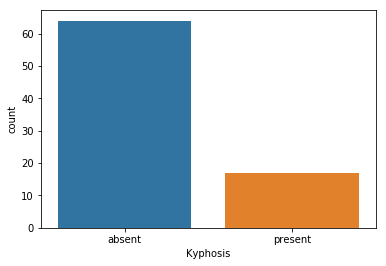In :
```from sklearn.preprocessing import LabelEncoder, OneHotEncoder
```
In :
```# change Kyphosis Column into number output
LabelEncoder_y = LabelEncoder()
kyphosis_df['Kyphosis'] = LabelEncoder_y.fit_transform(kyphosis_df['Kyphosis'])
```
In :
```kyphosis_df.head()
```
Out:
Kyphosis Age Number Start
0 0 71 3 5
1 0 158 3 14
2 1 128 4 5
3 0 2 5 1
4 0 1 4 15
In :
```kyphosis_true = kyphosis_df[kyphosis_df['Kyphosis']==1]
```
In :
```kyphosis_true.head()
```
Out:
Kyphosis Age Number Start
2 1 128 4 5
9 1 59 6 12
10 1 82 5 14
21 1 105 6 5
22 1 96 3 12
In :
```# 0 is usually equal to false
kyphosis_false = kyphosis_df[kyphosis_df['Kyphosis']==0]
```
In :
```kyphosis_false.head()
```
Out:
Kyphosis Age Number Start
0 0 71 3 5
1 0 158 3 14
3 0 2 5 1
4 0 1 4 15
5 0 1 2 16
In :
```print('Disease present after operation percentage is',
(len(kyphosis_true)/len(kyphosis_df))*100, '%')
```
```Disease present after operation percentage is 20.98765432098765 %
```
In :
```print('Disease not present after operation percentage is',
(len(kyphosis_false)/len(kyphosis_df))*100, '%')
```
```Disease not present after operation percentage is 79.01234567901234 %
```
In :
```sns.heatmap(kyphosis_df.corr(), annot = True)
```
Out:
`<matplotlib.axes._subplots.AxesSubplot at 0x1a151f24e0>`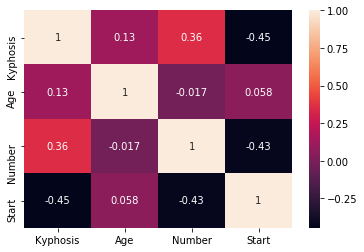In :
```sns.pairplot(kyphosis_df, hue='Kyphosis',
vars = ['Age', 'Number', 'Start'])
```
Out:
`<seaborn.axisgrid.PairGrid at 0x1a152e69b0>`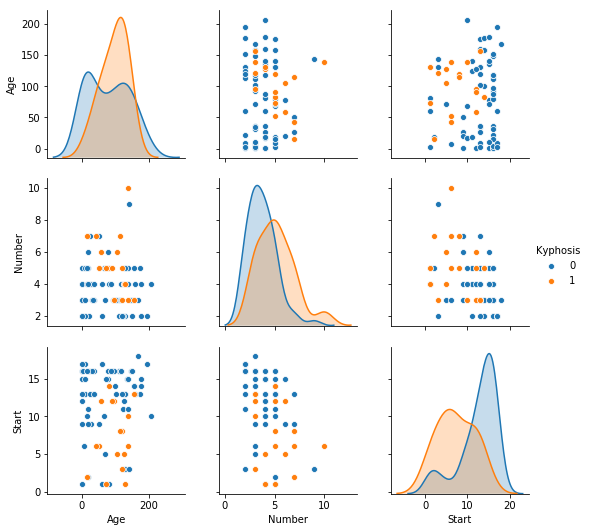### Data Prep¶

In :
```kyphosis_df.head()
```
Out:
Kyphosis Age Number Start
0 0 71 3 5
1 0 158 3 14
2 1 128 4 5
3 0 2 5 1
4 0 1 4 15
In :
```X = kyphosis_df.drop(['Kyphosis'], axis =1)
```
In :
```X.head()
```
Out:
Age Number Start
0 71 3 5
1 158 3 14
2 128 4 5
3 2 5 1
4 1 4 15
In :
```y = kyphosis_df['Kyphosis']
```
In :
```y.head()
```
Out:
```0    0
1    0
2    1
3    0
4    0
Name: Kyphosis, dtype: int64```
In :
```# Create train Test Split
from sklearn.model_selection import train_test_split
X_train, X_test, y_train, y_test = train_test_split(X,
y,
test_size=0.20,
random_state = 2,
stratify=y)
```

### Train Model¶

In :
```# Decision tree
from sklearn.tree import DecisionTreeClassifier
decision_tree = DecisionTreeClassifier()
decision_tree.fit(X_train, y_train)
```
Out:
```DecisionTreeClassifier(class_weight=None, criterion='gini', max_depth=None,
max_features=None, max_leaf_nodes=None,
min_impurity_decrease=0.0, min_impurity_split=None,
min_samples_leaf=1, min_samples_split=2,
min_weight_fraction_leaf=0.0, presort=False, random_state=None,
splitter='best')```
In :
```# Find feature importance
feature_importance = pd.DataFrame(decision_tree.feature_importances_,
index = X_train.columns,
columns = ['importance'] )
```
In :
```feature_importance
```
Out:
importance
Age 0.476436
Number 0.254160
Start 0.269404
In :
```# Sorted Feature Importance
feature_importance = pd.DataFrame(
decision_tree.feature_importances_,
index = X_train.columns,
columns = ['importance']).sort_values('importance',
ascending = False)
```
In :
```feature_importance
```
Out:
importance
Age 0.476436
Start 0.269404
Number 0.254160
In :
```from sklearn.metrics import confusion_matrix, classification_report
```
In :
```y_predict = decision_tree.predict(X_test)
```
In :
```cm = confusion_matrix(y_test, y_predict)
```
In :
```sns.heatmap(cm, annot =True)
```
Out:
`<matplotlib.axes._subplots.AxesSubplot at 0x1a15d50898>`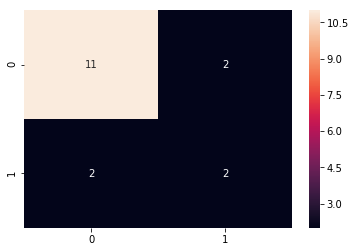In :
```print(classification_report(y_test, y_predict))
```
```             precision    recall  f1-score   support

0       0.85      0.85      0.85        13
1       0.50      0.50      0.50         4

avg / total       0.76      0.76      0.76        17

```
In :
```from sklearn.ensemble import RandomForestClassifier
```
In :
```randomforest_classifier = RandomForestClassifier(
n_estimators = 500,
criterion ='entropy')
```
In :
```randomforest_classifier.fit(X_train,y_train)
```
Out:
```RandomForestClassifier(bootstrap=True, class_weight=None, criterion='entropy',
max_depth=None, max_features='auto', max_leaf_nodes=None,
min_impurity_decrease=0.0, min_impurity_split=None,
min_samples_leaf=1, min_samples_split=2,
min_weight_fraction_leaf=0.0, n_estimators=500, n_jobs=1,
oob_score=False, random_state=None, verbose=0,
warm_start=False)```
In :
```y_predict_forest = randomforest_classifier.predict(X_test)
```
In :
```cm = confusion_matrix(y_test, y_predict_forest)
```
In :
```sns.heatmap(cm, annot = True)
```
Out:
`<matplotlib.axes._subplots.AxesSubplot at 0x1a162d40f0>`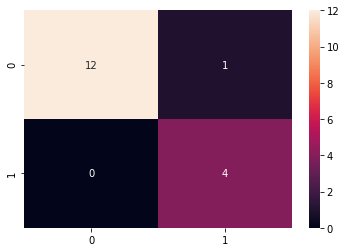In :
```print(classification_report(y_test, y_predict_forest))
```
```             precision    recall  f1-score   support

0       1.00      0.92      0.96        13
1       0.80      1.00      0.89         4

avg / total       0.95      0.94      0.94        17

```